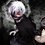# Need Help #Series

I was going through some proofathon problems and came over this: $\sum_{i=0}^\infty \sum_{j=0}^\infty \frac{1}{(2i)!(2j+1)!}$ . I know we have to use the exponential series in here. But I am still skeptical about my answer. I am getting my answer : $\frac{(e^{2}+1)(e^{2}-1)} {4e^{2}}$ . (Which I think is probably wrong. :P) Would be glad if someone would explain me how to approach such kind of problems. (I always stuck at double summation problems. :P)Note by Sanchit Ahuja
6 years, 3 months ago

This discussion board is a place to discuss our Daily Challenges and the math and science related to those challenges. Explanations are more than just a solution — they should explain the steps and thinking strategies that you used to obtain the solution. Comments should further the discussion of math and science.

When posting on Brilliant:

• Use the emojis to react to an explanation, whether you're congratulating a job well done , or just really confused .
• Ask specific questions about the challenge or the steps in somebody's explanation. Well-posed questions can add a lot to the discussion, but posting "I don't understand!" doesn't help anyone.
• Try to contribute something new to the discussion, whether it is an extension, generalization or other idea related to the challenge.
• Stay on topic — we're all here to learn more about math and science, not to hear about your favorite get-rich-quick scheme or current world events.

MarkdownAppears as
*italics* or _italics_ italics
**bold** or __bold__ bold
- bulleted- list
• bulleted
• list
1. numbered2. list
1. numbered
2. list
Note: you must add a full line of space before and after lists for them to show up correctly
paragraph 1paragraph 2

paragraph 1

paragraph 2

[example link](https://brilliant.org)example link
> This is a quote
This is a quote
    # I indented these lines
# 4 spaces, and now they show
# up as a code block.

print "hello world"
# I indented these lines
# 4 spaces, and now they show
# up as a code block.

print "hello world"
MathAppears as
Remember to wrap math in $$ ... $$ or $ ... $ to ensure proper formatting.
2 \times 3 $2 \times 3$
2^{34} $2^{34}$
a_{i-1} $a_{i-1}$
\frac{2}{3} $\frac{2}{3}$
\sqrt{2} $\sqrt{2}$
\sum_{i=1}^3 $\sum_{i=1}^3$
\sin \theta $\sin \theta$
\boxed{123} $\boxed{123}$

## Comments

Sort by:

Top Newest

Actually, double summation always doesn't involves applying some formula or some difficult series expansion. The real technique for solving most of the double summations lies in the fact that you need to recognize the series expansion. For this I would recommend to first have a good skills (an practice) of simple sums. Indeed learn to recognize series summations by practising more and more simple summation.

For example - the above sum can be evaluated easily if you remember that $e^x = \sum_{n=0}^\infty \frac{x^n}{n!}$

Now in the given sum, both $i$ and $j$ are independent variables, so we can write the given sum as

$\sum_{i=0}^\infty \sum_{j=0}^\infty \frac{1}{(2i)!(2j+1)!} = \left(\sum_{i=0}^\infty \frac{1}{(2i)!}\right) \left(\sum_{j=0}^\infty \frac{1}{(2j+1)!}\right)$

Now, the two sums are respectively the sum of reciprocal of even and odd factorials. TO find them we use the series expansion $e$ and $e^{-1}$

$e = \sum_{n=0}^\infty \frac{1}{n!}$

$e^{-1} = \sum_{n=0}^\infty \frac{(-1)^n}{n!}$

Adding both of them gives

$e+e^{-1} = 2\sum_{n=0}^\infty \frac{1}{(2n)!}$

Similarly subtracting the second one from first one gives

$e-e^{-1} = 2\sum_{n=0}^\infty \frac{1}{(2n+1)!}$

Thus, our required sum is $\sum_{i=0}^\infty \sum_{j=0}^\infty \frac{1}{(2i)!(2j+1)!} = \left(\frac{e+e^{-1}}{2}\right)\left(\frac{e-e^{-1}}{2}\right) = \frac{e^4-1}{4e^2}$

P.S. : The answer you have found is absolutely correct.

Therefore, as you see above I haven't used any advanced calculus methods to solve the above sum. Everything lies in just using basic techniques to simplify the sum and then use the results of well-known series.

Thanks,

Kishlaya Jaiswal.

- 6 years, 3 months ago

Log in to reply

Thanks @Kishlaya Jaiswal ! Help is appreciated! :D

- 6 years, 3 months ago

Log in to reply

It was all mine pleasure in explaining it. Also, I would encourage you to keep posting such interesting notes and feel free to ask for any help. $\ddot \smile$

- 6 years, 3 months ago

Log in to reply

Its correct. You can usually find answers to the Proofathon Contests on the website itself.

- 6 years, 3 months ago

Log in to reply

May be u should first master the single summation, then go for double summation. I think it needs calculus, and being an 11th class student mr.Ahuja, you are not aware of high level calculus. So try to deviate your focus towards basics first.

ALL THE BEST

- 6 years, 3 months ago

Log in to reply

FYI Mr. Bansal I did single summation and as stated above, it didn't involve any calculus. Thanks Mr. Bansal. For your "Valuable" piece of advice. All the best!

- 6 years, 3 months ago

Log in to reply

×

Problem Loading...

Note Loading...

Set Loading...